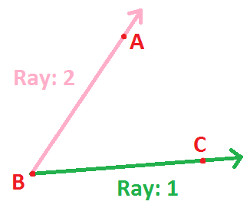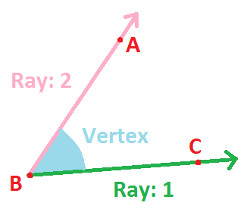Home >> Angles >>

Define Angle and Vertex

Exterior of Angles Interior of Angles Types of Angle Angle Construction (Compass) Angle Bisector (Compass) Congruent Angles

An Angle
When two rays meet at a single point An Angle is formed.
Below figure - shows that Ray 1 and Ray 2 meet at Point "O" and form An Angle AOB.Vertex
The point at which two Rays meet is known as Vertex of the Angle.
In the Below Figure, Ray 1 and Ray 2 meet at Point O, making it Vertex of Angle.